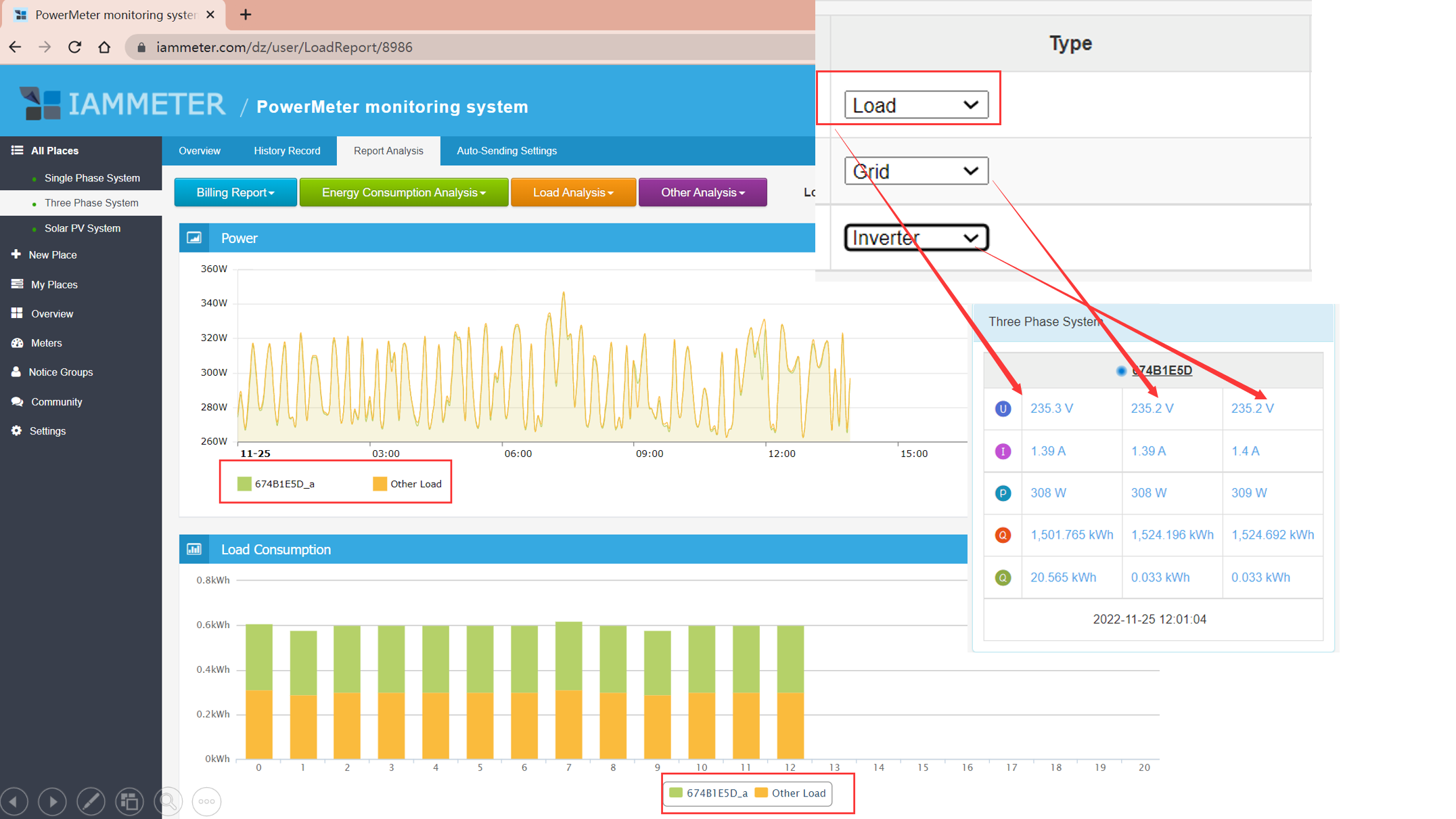### [New feature] Report:load consumption analysis

The aim of this report

Example explains

# The aim of this report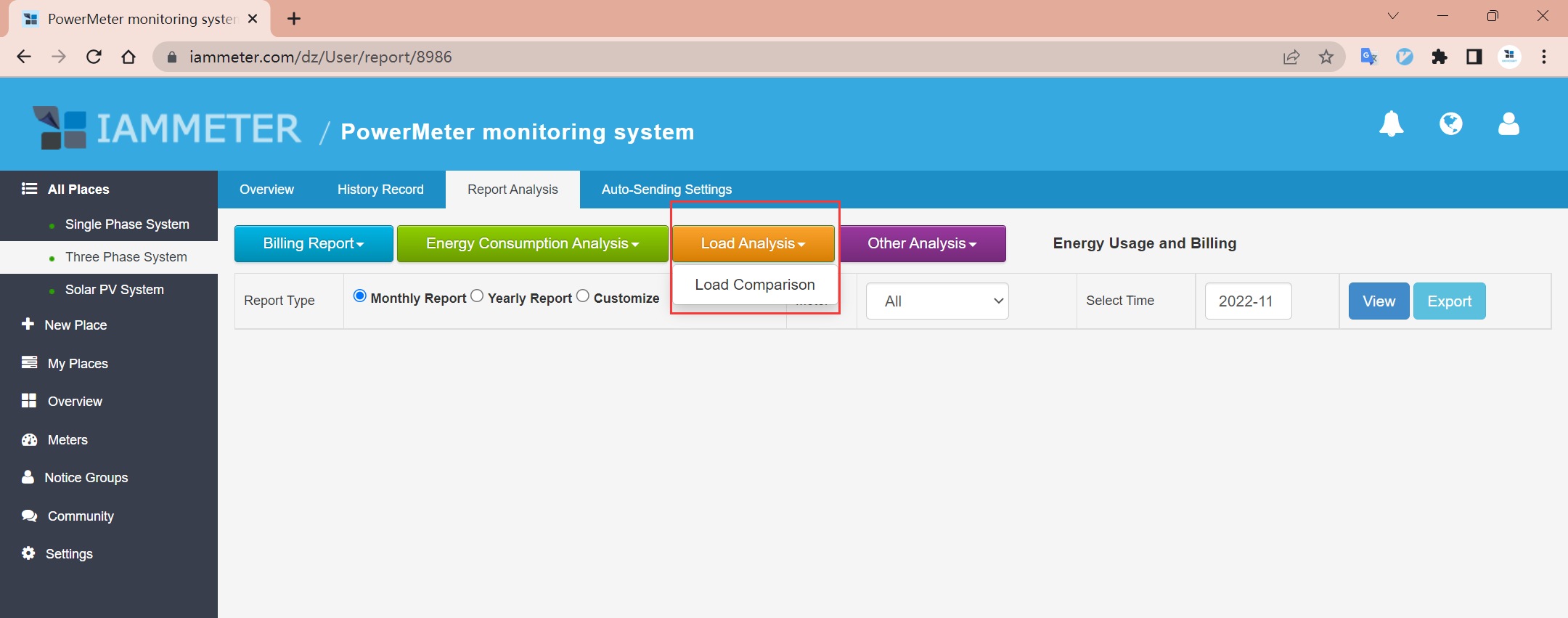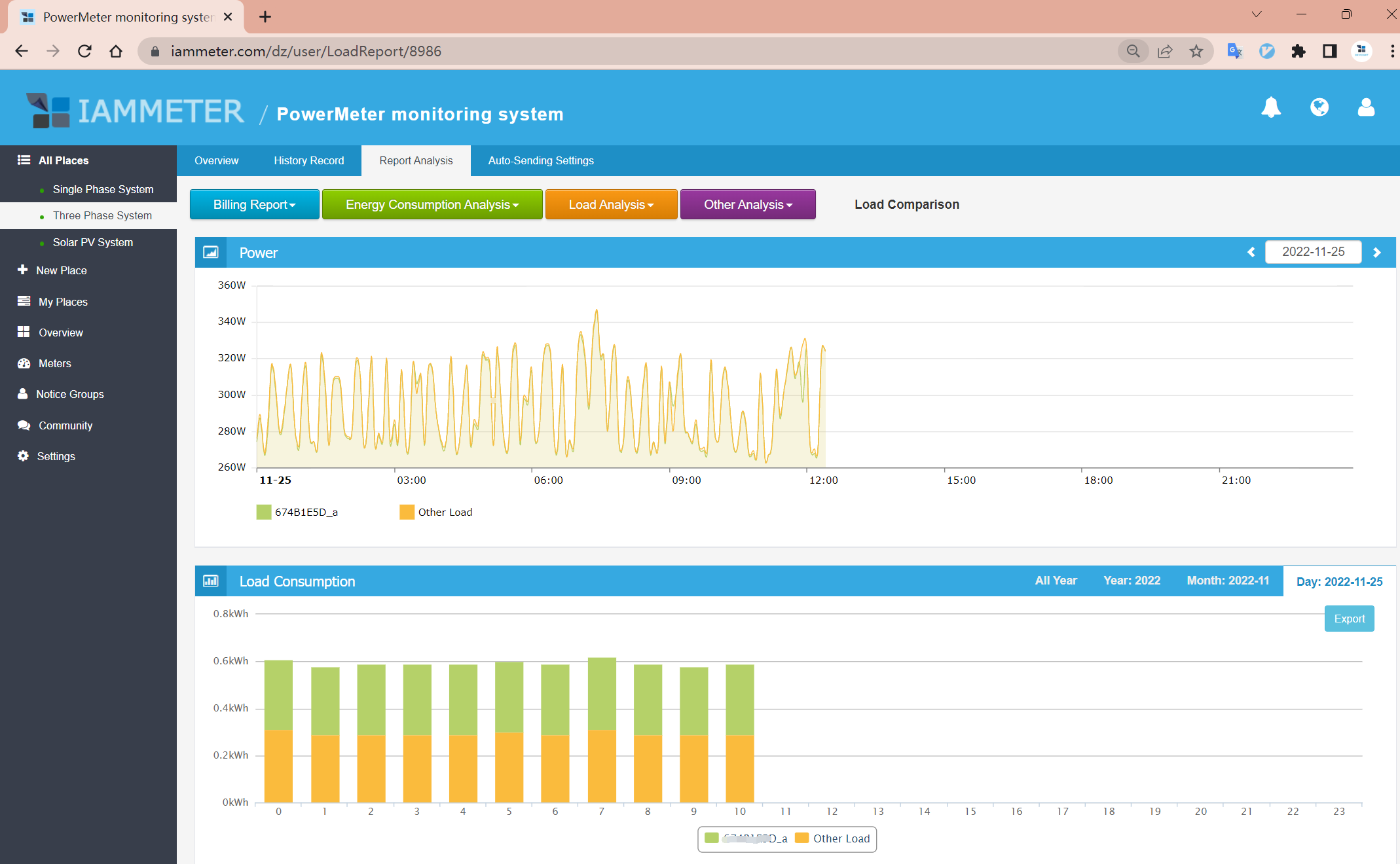# Precondition

• If you want to use this report ,there is at one meter type should be "load"(otherwise the "load Analysis" would not appear).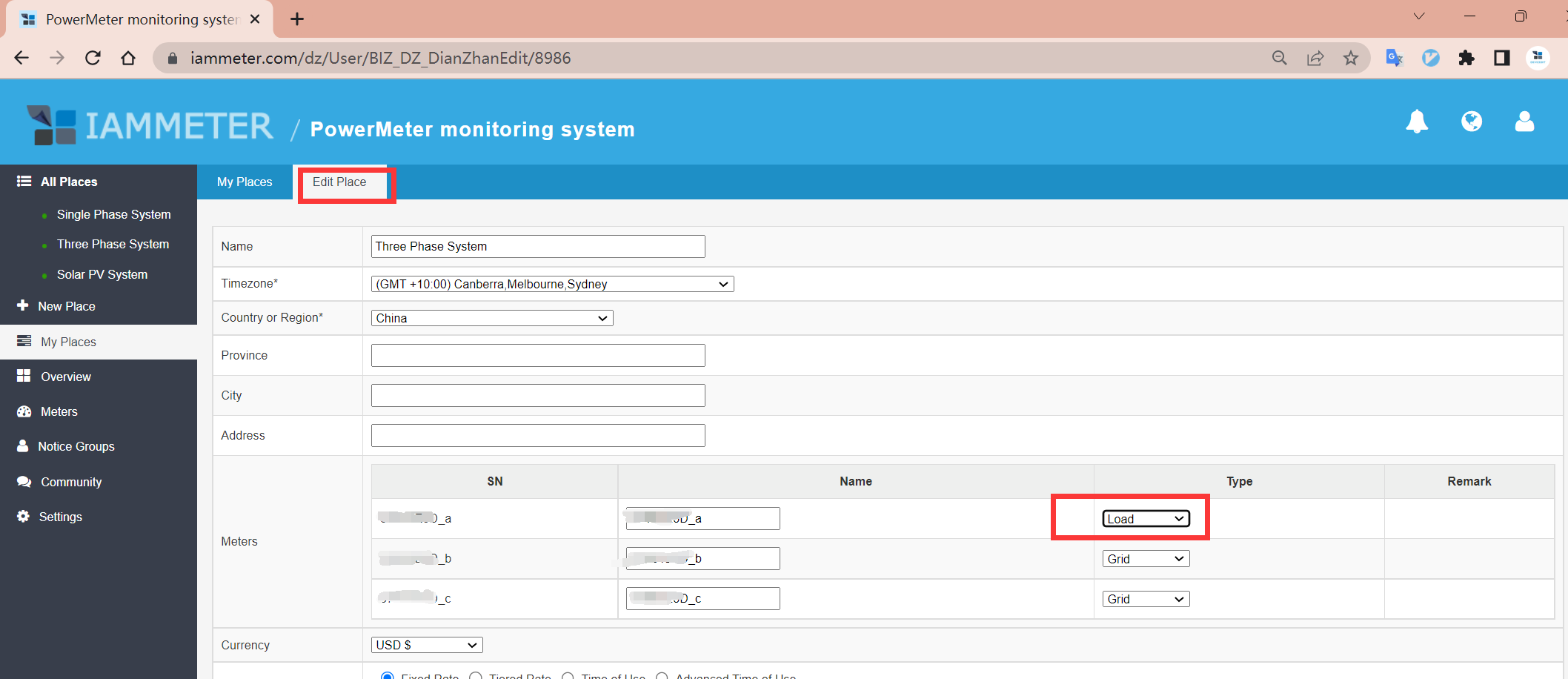# Example explain

For easy understanding, we use nearly the same 3phase input in the example. Just switch the meter type of each from "load" or "inverter".

`grid power = total load power -  solar inverter power total load power = special load power + Other load powerSpecial load :the phase that with meter type of "load"Other load: load except special load.`

## no inverter,only grid + load

In this case ,the "solar inverter power" = 0.

The grid power (sum phase B and phase C together) is nearly double the special load power(phase A).

So the other load power is nearly the same as the special load power.

The same logic existed in the kilowatt percent in the histogram graph.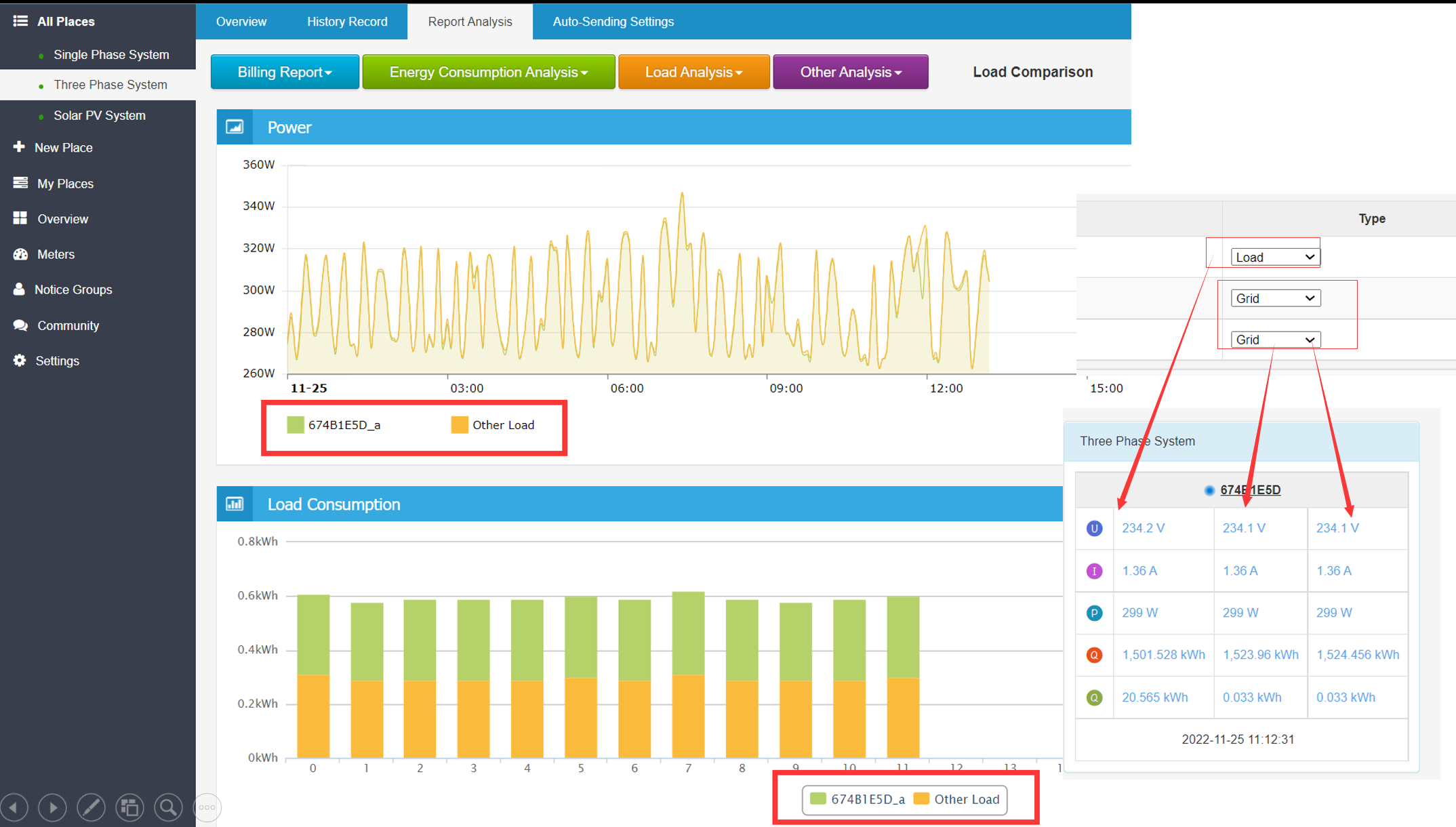In this case, the "solar inverter power" exists, and is also nearly the same as the grid power and load.

From the pic, you will find the report is also nearly the same as the above(which is no solar output), yes? let me make an explanation.

Let us review this formula again.

`grid power = total load power -  solar inverter power total load power = special load power + Other load power`

Because "solar inverter power "exists and is nearly the same as "grid power" either, the "total load power" (= grid power + solar inverter power) is also double of the "grid power".

So the "special load power" is nearly the half of the "total load power" either.